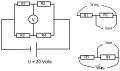# How to calculate voltage (V) between resistors in parallel?

#### DickCappels

Joined Aug 21, 2008
9,503
Probably not. You just have a 20 volt source and two voltage dividers. Just calculate the voltage across resistor in each divider, say the right side (R2 and R4) and then compare the voltages. It is hard to make it simpler than that.

#### Mannerheim

Joined Oct 16, 2021
4
Thank you,
to calculate voltage across resistor in each divider - is this the proper way to calculate:

R = (R2 * R4) / (R2 + R4)

R = (R1 * R4) / (R1 + R3)

#### n0dlb

Joined Oct 16, 2021
3
Hi. Don't overthink this. the 2 pairs of series resistors in parallel are still just basic voltage dividers. There is still 20V across both pairs.

Take series R1 + R2 10 ohm and 90 ohm, 100 ohm total so a 10/90 or 9:1 ratio, however you wanna look at it. So as a voltage divider you'll have 2V across R1 (10% of 20V and 18V on R2). By the same math 3V across R3 and 17V on R4.

Taking it a bit further what is the current in each leg?? not hard either, E=IR is all you need. I'll let YOU figure that out. You can then figure out the equivalent resistance with E=IR after you figure the current out, no need for fancy parallel resistance calculations. Then it's easy as P=IE to figure out the power!

#### n0dlb

Joined Oct 16, 2021
3
So sir...figure out the rest and post here...let us know if you get stuck

--Don N0DLB Don't look Back

#### Mannerheim

Joined Oct 16, 2021
4
Thank you for your kind help and suggestions. Basically the curcuit is a voltage divider (https://en.wikipedia.org/wiki/Voltage_divider) that can be divided into two linear voltage dividers.Vin = U = 20 V

Then the Vout from the first linear voltage divider is:
Vout1 = R2 x (Vin/(R1+R2))

The second:
Vout2 = R4 x (Vin/(R3+R4))

And to get the voltage between the two:

V = abs( Vout1 - Vout2 )

Last edited:

#### MrChips

Joined Oct 2, 2009
27,651

#### Mannerheim

Joined Oct 16, 2021
4
Because voltage is difference of potentials (Vout1 and Vout2). If Vout1 - Vout2 is a negative number, then the difference is absolute value of that negative number...

#### MrChips

Joined Oct 2, 2009
27,651
Because voltage is difference of potentials (Vout1 and Vout2). If Vout1 - Vout2 is a negative number, then the difference is absolute value of that negative number...
The negative sign is equally important as a positive sign. It has significance.
Don’t throw away the sign.

#### DickCappels

Joined Aug 21, 2008
9,503
For that to work, the solution needs to be the voltage on point A (for example) with respect to point B (also for example). The use of ABS is consistent with the problem as posed, but yes, it real life, the sign will usually be important.

#### BobaMosfet

Joined Jul 1, 2009
2,053
Hello,
I have added a picture about a simple circuit. I need to find Voltmeter reading between resistors as shown in picture.
The resistor values are:

R1 = 10
R2 = 90
R3 = 30
R4 = 170
Battery is 20 volts.
Is it possible to simplify the circuit?
View attachment 250454
Title: Understanding Basic Electronics, 1st Ed.
Publisher: The American Radio Relay League
ISBN: 0-87259-398-3

#### MrChips

Joined Oct 2, 2009
27,651
The negative sign is equally important as a positive sign. It has significance.
Don’t throw away the sign.
In an audio amplifier repair thread, we asked the TS to measure the voltage at a certain point in the circuit.
The TS failed to inform us that the voltage reading on the DMM was negative.
What a difference that negative sign made to the analysis of the circuit!

•DickCappels